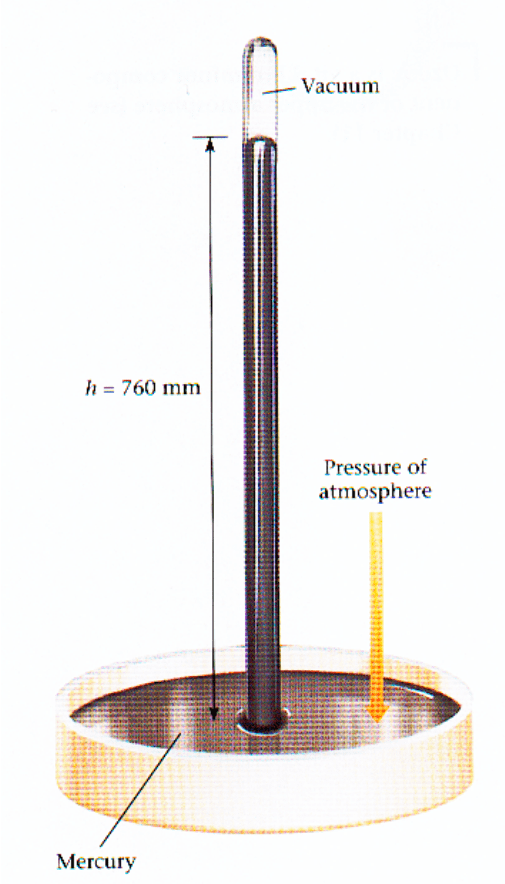Textbook Notes (270,000)
CA (160,000)
Western (10,000)
CHEM (200)
Chapter 3

Chemistry 1024A/B Chapter 3: Chem1024b_2013_chapter03-1.pdf

Department
Chemistry
Course Code
CHEM 1024A/B
Professor
Robert Klassen
Chapter
3

This preview shows pages 1-3. to view the full 24 pages of the document.75
Chapter 3: Gases
3.1 Measurements on Gases MHw, Chapter 5.1
Let’s look at a certain amount of gas, e.g. trapped
inside a balloon. To completely describe the state of
this gas one has to specify the following quantities:
Volume
Symbol: V
Units: L 1 L = 10-3 m3
(remember that L is not a SI unit)
Temperature
Symbol: T
Units: K (“Kelvin”). The Kelvin scale is the “absolute
temperature” scale.
To convert ° C to K we simply add 273
(actually it is 273.15, but in most cases “273” will do)
Example: 25° C = (25 + 273) K = 298 K
Pressure
Symbol: p
Units: force per area 1Pa (Pascal) = 1 N/m2
Other units are still used for pressure:
105 Pa = 1 bar
101300 Pa = 101.3 kPa = 1.013 bar = 1 atm = 760 mm Hg = 760 Torr
psi: pound per square inch (we will avoid that; 1 psi = 6.894 kPa)
MHw mostly uses atm, we will use kPa, to be
consistent across Chem1050 / 1024.
Remember: 1 atm = 101.3 kPa

Only pages 1-3 are available for preview. Some parts have been intentionally blurred.76
A mercury barometer can be used for measuring gas
pressure
“Normal pressure” corresponds to 101.3 kPa = 760
mm Hg ( =1 atm).
The Hg level will be
lower when you move
this device from the
basement to the third
floor, or when a
rainstorm is moving in
(why?)
MHw, Figure 5.1

Only pages 1-3 are available for preview. Some parts have been intentionally blurred.77
Number of Moles
With gases, like with any other substances we can
use the relationship
m = MM × n
to convert mass (m) to number of moles (n), if we
know the molar mass (MM).
Example: How many moles of N2 molecules are there
in 2.50 g?
Calculations involving gases can be confusing
because of the different units that are sometimes
used for the same quantity.
That means: before you do any calculations
Convert the volume to L
Convert the pressure to kPa
Convert the temperature to K
Convert the “amount” of material to moles
and you’ll be OK !